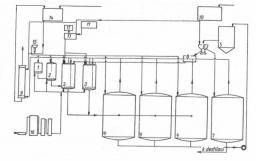# Solution 3092

How many liters of 80% alcohol need to be mixed with 4 liters of 40% alcohol to get 70% alcohol? How many liters of 70% solution do we get?

x =  12
y =  16

### Step-by-step explanation:

0.80 x + 0.40•4 = 0.70 • y
y = x+4

0.80•x + 0.40•4 = 0.70 • y
y = x+4

0.8x-0.7y = -1.6
x-y = -4

x = 12
y = 16

Our linear equations calculator calculates it.Did you find an error or inaccuracy? Feel free to write us. Thank you!

Tips for related online calculators